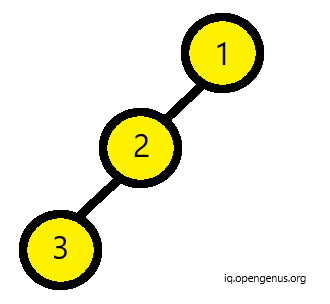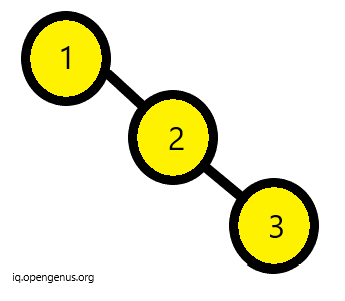×

Search anything:

# Introduction to Skewed Binary Tree

#### Problems on Binary Tree AlgorithmsA binary tree can be called a skewed binary tree if all nodes have one child or no child at all. They can be of two types:

• Left Skewed Binary Tree
• Right Skewed Binary Tree

## Left Skewed Binary Tree

If all nodes are having a left child or no child at all then it can be called a left skewed binary tree. In this tree all children at right side remain null.## Right Skewed Binary Tree

If all nodes are having a right child or no child at all then it can be called a right skewed binary tree. In this tree all children at left side remain null.## Implementation of Binary Tree

``````//definition of a node
struct node{
int value;
struct node *left,*right;
}
//function to make new node
node* newnode(int value){
node* t = new node;
t->value = value;
t->left = t->right = Null;
return(t);
}
``````

## Case 1 : Left skewed binary tree

In case of left skewed binary tree, we will insert values only in left nodes and leave all right nodes as null.

``````node * root= newnode(5);
root->left = newnode(8);
root->left->left = newnode(10);
``````

The above code snippet creates a new node, that will be the root for our tree and initialises it with value 5. Then, it creates its left node with value 8 and further its left node witrh value 10.

## Case 2 : Right skewed binary tree

In case of rigth skewed binary tree, we will insert values only in right nodes and leave all left nodes as null.

``````node * root= newnode(5);
root->right = newnode(8);
root->right->right = newnode(10);
``````

The above code snippet creates a new node, that will be the root for our tree and initialises it with value 5. Then, it creates its right node with value 8 and further its right node witrh value 10.

This is the simple implementation for the skewed binary tree.
Happy Coding!#### Srishti Guleria

Srishti Guleria has been a Technical Author, Software Developer, Intern at OpenGenus and a Computer Science student at Rajasthan Technical University, Kota

Introduction to Skewed Binary Tree